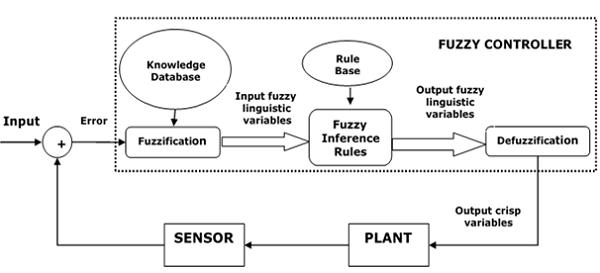# Fuzzy Logic - Control System

Fuzzy logic is applied with great success in various control application. Almost all the consumer products have fuzzy control. Some of the examples include controlling your room temperature with the help of air-conditioner, anti-braking system used in vehicles, control on traffic lights, washing machines, large economic systems, etc.

## Why Use Fuzzy Logic in Control Systems

A control system is an arrangement of physical components designed to alter another physical system so that this system exhibits certain desired characteristics. Following are some reasons of using Fuzzy Logic in Control Systems −

• While applying traditional control, one needs to know about the model and the objective function formulated in precise terms. This makes it very difficult to apply in many cases.

• By applying fuzzy logic for control we can utilize the human expertise and experience for designing a controller.

• The fuzzy control rules, basically the IF-THEN rules, can be best utilized in designing a controller.

## Assumptions in Fuzzy Logic Control (FLC) Design

While designing fuzzy control system, the following six basic assumptions should be made −

• The plant is observable and controllable − It must be assumed that the input, output as well as state variables are available for observation and controlling purpose.

• Existence of a knowledge body − It must be assumed that there exist a knowledge body having linguistic rules and a set of input-output data set from which rules can be extracted.

• Existence of solution − It must be assumed that there exists a solution.

• ‘Good enough’ solution is enough − The control engineering must look for ‘good enough’ solution rather than an optimum one.

• Range of precision − Fuzzy logic controller must be designed within an acceptable range of precision.

• Issues regarding stability and optimality − The issues of stability and optimality must be open in designing Fuzzy logic controller rather than addressed explicitly.

## Architecture of Fuzzy Logic Control

The following diagram shows the architecture of Fuzzy Logic Control (FLC).## Major Components of FLC

Followings are the major components of the FLC as shown in the above figure −

• Fuzzifier − The role of fuzzifier is to convert the crisp input values into fuzzy values.

• Fuzzy Knowledge Base − It stores the knowledge about all the input-output fuzzy relationships. It also has the membership function which defines the input variables to the fuzzy rule base and the output variables to the plant under control.

• Fuzzy Rule Base − It stores the knowledge about the operation of the process of domain.

• Inference Engine − It acts as a kernel of any FLC. Basically it simulates human decisions by performing approximate reasoning.

• Defuzzifier − The role of defuzzifier is to convert the fuzzy values into crisp values getting from fuzzy inference engine.

## Steps in Designing FLC

Following are the steps involved in designing FLC −

• Identification of variables − Here, the input, output and state variables must be identified of the plant which is under consideration.

• Fuzzy subset configuration − The universe of information is divided into number of fuzzy subsets and each subset is assigned a linguistic label. Always make sure that these fuzzy subsets include all the elements of universe.

• Obtaining membership function − Now obtain the membership function for each fuzzy subset that we get in the above step.

• Fuzzy rule base configuration − Now formulate the fuzzy rule base by assigning relationship between fuzzy input and output.

• Fuzzification − The fuzzification process is initiated in this step.

• Combining fuzzy outputs − By applying fuzzy approximate reasoning, locate the fuzzy output and merge them.

• Defuzzification − Finally, initiate defuzzification process to form a crisp output.

## Advantages of Fuzzy Logic Control

Let us now discuss the advantages of Fuzzy Logic Control.

• Cheaper − Developing a FLC is comparatively cheaper than developing model based or other controller in terms of performance.

• Robust − FLCs are more robust than PID controllers because of their capability to cover a huge range of operating conditions.

• Customizable − FLCs are customizable.

• Emulate human deductive thinking − Basically FLC is designed to emulate human deductive thinking, the process people use to infer conclusion from what they know.

• Reliability − FLC is more reliable than conventional control system.

• Efficiency − Fuzzy logic provides more efficiency when applied in control system.

## Disadvantages of Fuzzy Logic Control

We will now discuss what are the disadvantages of Fuzzy Logic Control.

• Requires lots of data − FLC needs lots of data to be applied.

• Useful in case of moderate historical data − FLC is not useful for programs much smaller or larger than historical data.

• Needs high human expertise − This is one drawback as the accuracy of the system depends on the knowledge and expertise of human beings.

• Needs regular updating of rules − The rules must be updated with time.